# Seismic stressesIntroduction >
The structural frame
The construction
The reinforcement I
The reinforcement II
Quantity/Cost estimation
Detailing drawingsIntroduction >
Wind and Seismic Forces >
Structural model and Analysis
Modelling slabs
Slabs
Seismic behavour of frames
Appendix A
Appendix B
Appendix C
Appendix DIntroduction >
Materials
To be continued >

## Seismic accelerations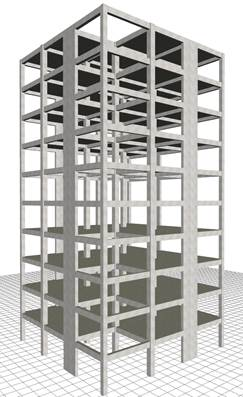Seismic action in X direction Mass a/g H[kN] V[kN]Ten-storey building of mixed system. Diaphragmatic and non-diaphragmatic nodes. Project Page from the software report Distribution of seismic accelerations-forces-shear forces

The seismic accelerations aXX, aXY, aXZ of each diaphragm are obtained under the X horizontal component of the seismic action using appropriate methods (e.g. the CQC method applied by the related software) from the combination of the modal analysis results. Their application point of the seismic accelerations is the diaphragm’s centre of mass. For nodes not belonging to a diaphragm, such as the nodes of the corner column of the building illustrated in the figure, the application point of the seismic accelerations is the node position.

## Applications: Frame type structureStructure comprising only columns, project <B_641-1>

Four cases are considered:

1. Fixed condition at the ground level
2. Foundation with footing beams
3. Foundation with simple footings
4. Basement with perimeter walls

The stiffnesses of elements are taken being equal to 50% of elastic. 12 mode shapes are taken into account.

## General conclusions of the frame system

1)    In all cases only the x horizontal component of the seismic action is considered, the y component produces almost identical results.

2)    The natural period of the first mode shape is of the order of 1 sec, while, if the stiffnesses of the elements are taken as being 100% of the elastic, the value of periods is of order of 0.65 sec (see §6.3.3).

3)    Apparently the more economical solution of simple footings without strap (connecting) beams eventually becomes the most unfavourable one, even for economic reasons due to the need for additional reinforcement and increased dimensions of beams and columns cross-sections.

4)    The best solution is the basement with perimeter walls, i.e. along the 75% of the perimeter, as providing behaviour that better approximates the fixed condition.

5)   The bending moments of the two characteristic columns reflect the stress relation between the various cases.

## Modal analysis results of the frame type structure Fixed condition at the ground level (project <Β_641-1>)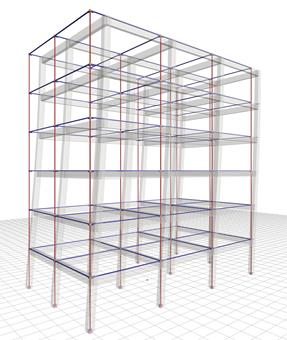Figure 6.4.1-2: 1st mode in X: T=0.975 sec, participation 84% Figure 6.4.1-3: 2nd mode in X: T=0.321 sec, participation 10%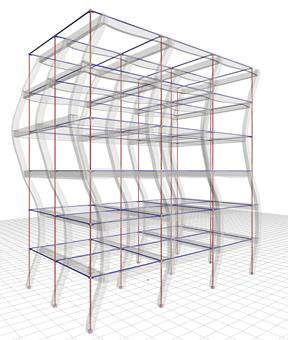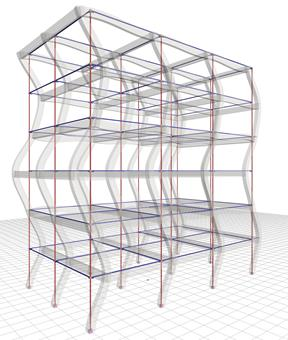Figure 6.4.1-4: 3rd mode in X: T=0.189 sec, participation 3.5% Figure 6.4.1-5: 4th mode in X: T=0.134 sec, participation 1.6%

The sum of the effective modal masses of the first four modes amounts to 99% of the total mass. The first mode is the fundamental one as its effective mass is the 84% of the total mass.

## Case 1: Fixed condition at the ground level (project <Β_641-1>)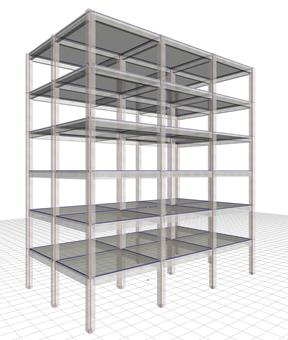Figure 6.4.1-6: Structure and model Frame system with q=3.60 Figure 6.4.1-7: Seismic acceleration-forces-shear forces 1st fundamental period:T1=0.975 sec, participation 84%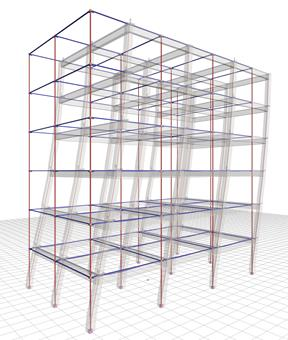Bending moments of ground floor columns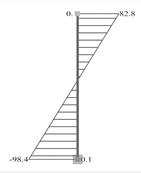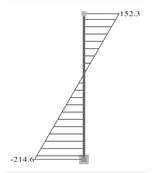Figure 6.4.1-8: Displacements under seismic action in x δmax=24.5 mm Figure 6.4.1-9: Ground floor column 0c2 (400/400) Figure 6.4.1-10: Ground floor column 0c6 (500/500)
The bending moment of the middle column c6 is roughly twice the value corresponding to the perimeter column, due to its stronger section.

## Case 2: Foundation with footing beams (project <Β_641-2>)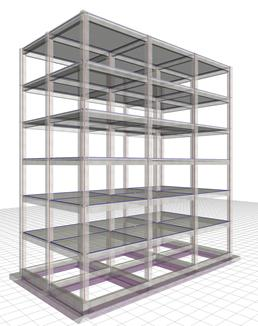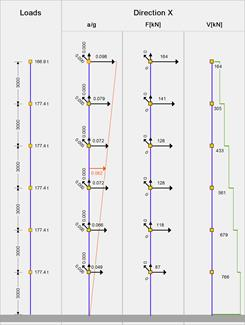Figure 6.4.1-11: Structure and model Frame system with q=3.60 Figure 6.4.1-12: Seismic acceleration-forces-shear forces 1st fundamental period:T1=1.012 sec, participation 85%Bending moments of ground floor columns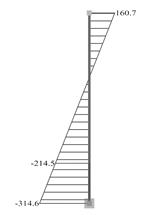Figure 6.4.1-13: Displacements under seismic action in x δmax=25.7 mm Figure 6.4.1-14: Ground floor column 0c2 (400/400) Figure 6.4.1-15: Ground floor column 0c6 (500/500)

Due to stronger cross-sections of footing beams compared to those of columns, the displacement of the structure is slightly larger than that of assumed fixity at the base (25.7 against 24.5).

## Case 3: Foundation with simple footings (project <Β_641-3>)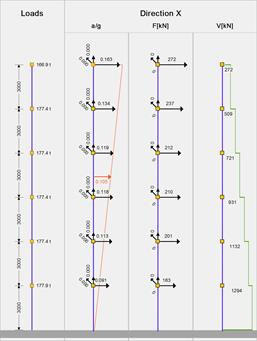Figure 6.4.1-16: Structure and model Torsionally flexible system with q=2.00 Figure 6.4.1-17: Seismic acceleration-forces-shear forces 1st fundamental period:T1=1.075 sec, participation 88%Bending moments of ground floor columns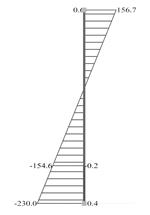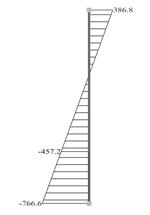Figure 6.4.1-18: Displacements under seismic action in x δmax=47.6 mm Figure 6.4.1-19: Ground floor column 0c2 (400/400) Figure 6.4.1-20: Ground floor column 0c6 (500/500)

In the absence of strap beams, the structure is classified as torsionally flexible system with q=2.00.

The displacement is almost twice that of the strong foundation (47.6 against 25.7 mm).

## Case 4: Basement with perimeter walls (project <Β_641-4>)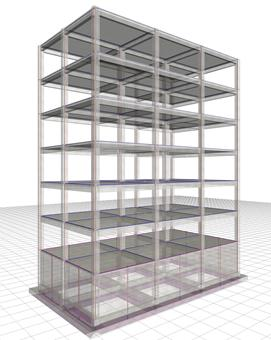Figure 6.4.1-21: Structure and model Frame system with q=3.60 Figure 6.4.1-22: Seismic acceleration-forces-shear forces 1st fundamental period:T1=0.999 sec, participation 71%Bending moments of ground floor columns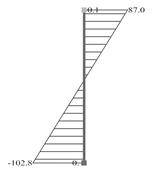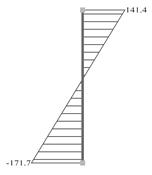Figure 6.4.1-23: Displacements under seismic action in x δmax=25.3 mm Figure 6.4.1-24: Ground floor column 0c2 (400/400) Figure 6.4.1-25: Ground floor column 0c6 (500/500)

The overall behaviour of the structure is better than the one corresponding to the strong foundation at the ground floor, approaching that for assumed fixity at the base.

## Applications: Wall-equivalent dual type structure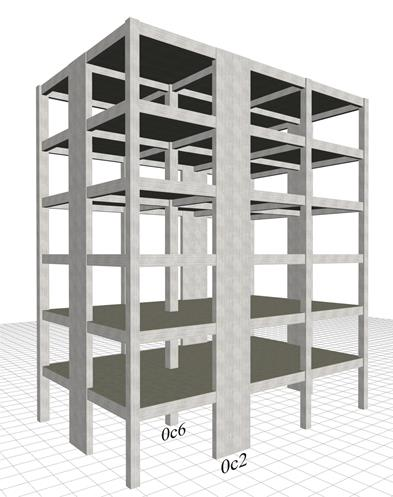Structure comprising only columns and perimeter walls, project <B_642-1>

The structure derives from the frame type structure by replacing columns c2, c5, c8 and c11 of cross-section 400/400 with four perimeter walls of cross-section 2000/300.

It is extremely useful to compare between the variants of the wall system, but also between the frame and wall systems.

## General conclusions of the wall system

1)    In all cases, the natural period of the first mode shape is of the order of 0.70 sec. If however the stiffnesses of the elements are taken as being 100% of the elastic, the value of periods is of order of 0.50 sec.

2)     The wall system behaviour is clearly better than the behaviour of the frame system, particularly when in the presence of a basement with perimeter walls.

## Modal analysis results of the wall type structure Fixed condition at the ground level (project <Β_642-1>)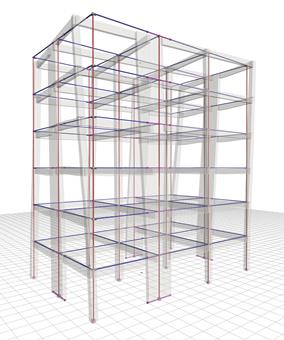Figure 6.4.2-2: 1st mode: Tx=0.644 sec, Cx=6.6%, Cy=70.7% Figure 6.4.2-3: 2nd mode: Tx=0.639 sec, Cx=70.5%, Cy=6.6%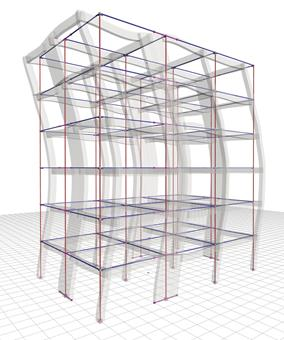Figure 6.4.2-4: 3rd mode: Tx=0.456 sec, Cx=0.5%, Cy=0.0% Figure 6.4.2-5: 4th mode: Tx=0.187 sec, Cx=1.8%, Cy=10.7%

In the absence of symmetry in y direction, the fundamental mode shape in x (the 2nd) has also a component in y, meaning that both translational and torsional responses are developed.

The sum of the effective modal masses of the 12 first modes amounts to 99.5% and 98.1% of the total mass of the structure in x and in y directions respectively.

## Case 1: Fixed condition at the ground level (project <Β_642-1>)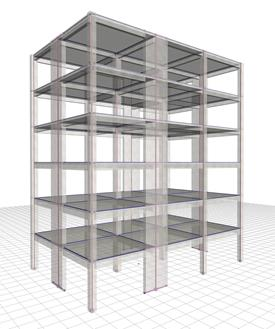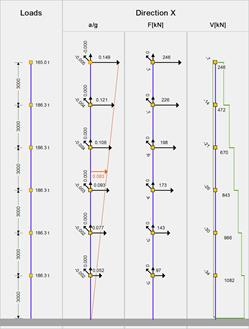Figure 6.4.2-6: Structure and model Ductile wall system in X and Y, with q=3.60 Figure 6.4.2-7: Seismic acceleration-forces-shear forces 1st fundamental period:T1X=0.639 sec, participation 70%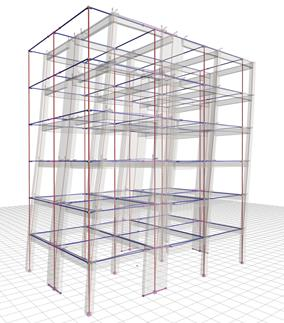Bending moments of ground floor columns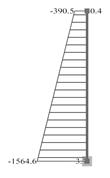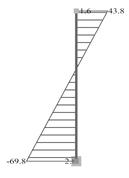Figure 6.4.2-8: Displacements under seismic action in x δmax=16.3 mm Figure 6.4.2-9: Ground floor wall 0c2 (2000/300) Figure 6.4.2-10: Ground floor column 0c6 (500/500)

The displacement of the wall type structure is significantly smaller than the one of the frame type structure of the previous paragraph (16.3 mm against 24.5 mm).

The bending moment of the first storey, is carried almost exclusively by the walls.

## Case 2: Foundation with footing beams (project <Β_642-2>)Figure 6.4.2-11: Wall-equivalent dual system in X, ductile wall system in Y, with Y, q=3.00 Figure 6.4.2-12: Seismic acceleration-forces-shear forces 1st fundamental period:T1X=0.709 sec, participation 75%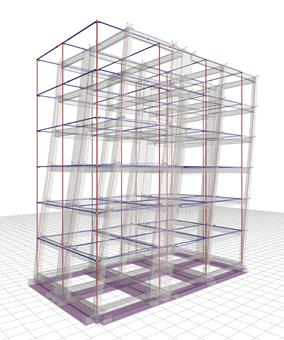Bending moments of ground floor columns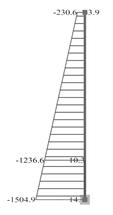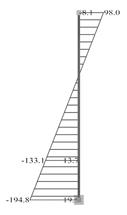Figure 6.4.2-13: Displacements under seismic action in x δmax=21.3 mm Figure 6.4.2-14: Ground floor wall 0c2 (2000/300) Figure 6.4.2-15: Ground floor column 0c6 (500/500)

The cross-sections of the footing beams are not strong enough to carry the high bending moments of the perimeter walls, resulting in the system along y to shift to a wall-equivalent dual system one with overall q=3.00. Moreover, the displacement of this system, along x, is equal to 21.3 mm compared to 25.7 mm of the corresponding frame system. Compared to case 1, the bending moments of the walls are smaller, while of columns are higher.

## Case 3: Foundation with simple footings (project <Β_642-3>)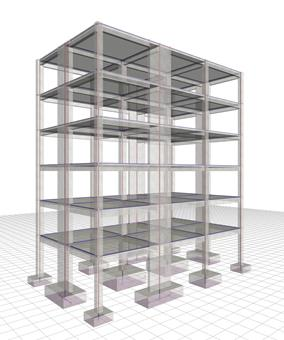Figure 6.4.2-16: Structure and model Wall-equivalent dual system in X and Y with q=3.00 Figure 6.4.2-17: Seismic acceleration-forces-shear forces 1st fundamental period:T1X=0.818 sec, participation 78%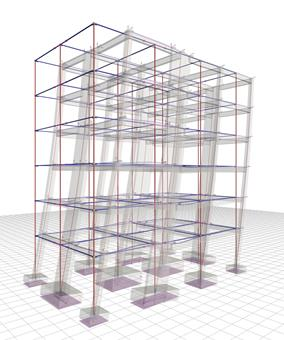Bending moments of ground floor columns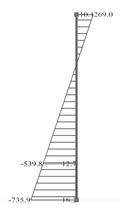Figure 6.4.2-18: Displacements under seismic action in x δmax=23.9 mm Figure 6.4.2-19: Ground floor wall 0c2 (2000/300) Figure 6.4.2-20: Ground floor column 0c6 (500/500)

In both directions the structure functions as a wall-equivalent dual system, due to attenuation of wall behaviour caused by the ground’s low ability to oppose rotation.

The situation would be considerably improved using strong strap beams.

The bending moments of the first storey walls reflect more the frame than the wall behaviour.

## Case 4: Basement with perimeter walls (project <Β_642-4>)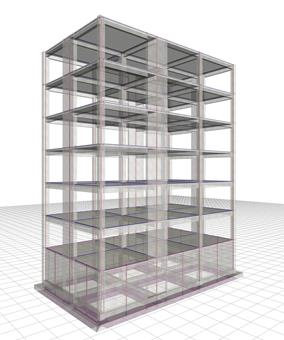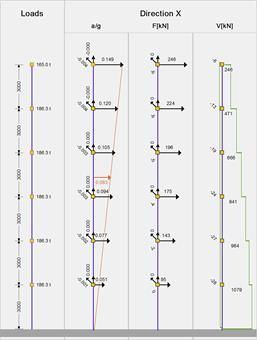Figure 6.4.2-21: Structure and model Ductile wall system in X and Y with q=3.60 Figure 6.4.2-22: Seismic acceleration-forces-shear forces 1st fundamental period:T1X=0.643 sec, participation 61%Bending moments of ground floor columns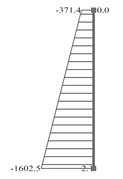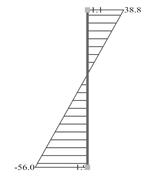Figure 6.4.2-23: Displacements under seismic action in x δmax=16.4 mm Figure 6.4.2-24: Ground floor wall 0c2 (2000/300) Figure 6.4.2-25: Ground floor column 0c6 (500/500)

## Approximate analysis of the frame type structure

Step 1:

The building height is H=6·3.0=18.0 m.

The structure is classified as wall system in both directions, thus Ct,x=Ct,y=Ct=0.050. The structure is also regular in both plan and elevation, thus q=3.60.

T1,x= T1,y=Τ1=Ct·H3/4=0.050·18.03/4=0.44 sec

Step 2:

Since 0.15=TB≤0.44=T1<0.50=TC (see §6.1.6)
Sd(T1)=γI·agR·S·(2.5/q)=1.0·0.15·1.2·2.5/3.6= 0.125g.

Since T1=0.44≤1.00=2TC à λ=0.85, thus aCM=λ·Sd(T1)=0.85·0.125g=0.10625g

Step 3:

The mass of each floor is M=186.3 t, except from the mass of the last floor which is M6=165.0 t, therefore Σ(Mi)=165.0+5·186.3=1096.5 t.

The elevation of the centre of mass is

ZCM=Σ(Mj·Zj)/Σ(Mj)= =(186.3·3.0+186.3·6.0+186.3·9.0+186.3·12.0+186.3·15.0+165.0·18.0)/1096.5=11353.5/1096.5 à ZCM=10.4 m

The triangular distribution gives the accelerations by means of the expression

aj=(Zj/ZCM)·aCM=(0.10625g/10.4)·Zj à aj=0.0102g·Zj

which yields a1=0.031g, a2=0.061g, a3=0.092g, a4=0.122g, a5=0.153g, a6=0.184g and seismic forces H1=a1·M1=0.031·10m/sec2·186.3·103kg=58 kN, H2=114, H3=171, H4=227, H5=285 and H6=0.184·10m/sec2·165·103kg =304 kN.

## Conclusions

a)    The value of the approximate calculation of the fundamental natural period and the design seismic acceleration lies in checking the correctness of the corresponding quantities derived from the algorithms of the modal response spectrum analysis.

In the related software, the fundamental natural period is obtained from the modal response spectrum analysis and is shown as a red arrow in the seismic acceleration graph at the elevation of the centre of mass.

b)    The value of the approximate method for the triangular distribution of seismic accelerations consists in the evaluation of the accelerations obtained by the algorithms of the modal response spectrum analysis, which should follow the triangular distribution to some extent.

In the related software, the triangular distribution is shown using a red dashed line.